Practice the problems of Math in Focus Grade 5 Workbook Answer Key Chapter 8 Decimals to score better marks in the exam.

Math Journal

Question 1.
Explain why 1.8, 1.80, and 1.800 have the same value.
Answer: 1.8, 1.80, and 1.800 have the same value as all the three values have 0 at their decimal value after 8.
since the value after the decimal point if it’s less than 5 then the value will be the same and there won’t be much change

Question 2.
Howard does not know how to find the values of A and B on the number line. Write the steps Howard should use to find these values.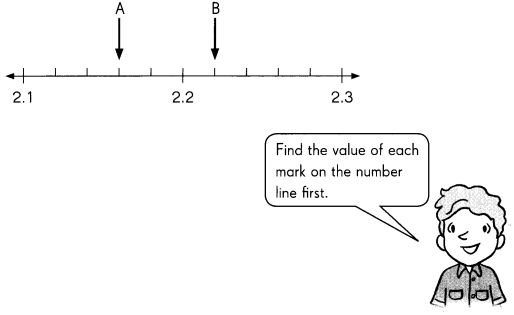Howard should use the tenth and hundredth value counting and then rounding value  method to identify the value
that is to find A Howard should start counting numbers from2.1 and go on as 2.11,2.12,2.13,2.14,2.15,2.16 at 2.16 the value is hundredth value is more than 5 so the value will be 2.2
and at the point A value will be 2.13
Same to find value of B it will be 2.21

Challenging Practice

Solve.

Question 1.
You are given two numbers, 3.987 and 70.140.

a. Round each number to the nearest tenth.
The nearest tenth for 3.987 is 4.0
The nearest tenth for 70.140 is 70.1
Explanation:
For 3.987,  the nearest tenth place value is 9 and to round the number which is nearest to the tenth we have to look at the next place value which is the hundredth so 8 is more than 5 we have to go onto the next highest tenth value  will be 4.o
For 70.140,  the nearest tenth place value is 1 and to round the number which is nearest to the tenth we have to look at the next place value which is the hundredth so since 4 is less than 5 the round of value will be 70.1

b. Round each number to the nearest hundredth.
Explanation:
The nearest hundredth for 3.987 is 3.99
The nearest hundredth for 70.140 is 70.14
For 3.987, to get to the nearest hundredth we go to the thousandth position and the value is more than  5 we have to go onto the next highest hundredth value which will be 3.99
For 70.140,  the nearest hundredth place value is 4 and to round the number which is nearest to the hundredth we have to look at the next place value which is the thousandth so since 0 is less than 5 the round of value will be 70.14

The nearest tenth for 3.987 is 4.0
The nearest hundredth  for 3.987 is 3.99
By removing 3.99 from 4.0 we get 0.01
4.0 – 3.99 = 0.01
so, The difference will be 0.01

The nearest tenth for 70.140 is 70.1
The nearest hundredth  for 70.140 is 70.14
By removing 70.1 from 70.14 we get 0.04
70.14 – 70.1 = 0.04
so, The difference will be 0.04

e. Are your answers in Exercises a and b the same? Explain why or why not.
Answer: No, the answers are not same as the exercises a is done to find the round value of tenth and the exercise b is done to find the round value of hundredth.

Complete.

Question 2.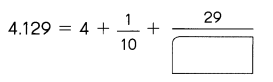4.129=4+0.1+0.029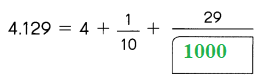Question 3.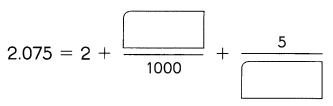2.075=2+0.070+0.005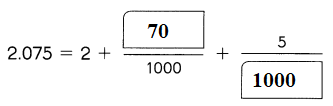Question 4.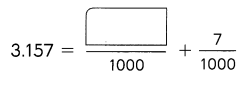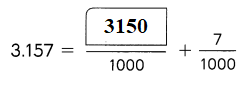Problem Solving

Question 1.
Kimberly has 3.25 kilograms of flour in a container. She adds 45 grams of flour to the container. How many kilograms of flour does she have now?
Kimberly has 3.25 kilograms of flour in a container.
Kimberly has 3.25 kilograms of flour in a container.
Convert grams into kilograms
To convert grams divide 45 with 1000
As 1 gram is equal to 0.001 kilogram
45 grams = $$\frac{45}{1000}$$
= 0.045  Kilograms
Now by adding 3.25 and 0.045 we get 3.295 kilogram
3.25 + 0.045 = 3.295 kg

Question 2.
The weight of four objects are 3$$\frac{1}{5}$$ pounds, 3$$\frac{39}{1000}$$ pounds, 3$$\frac{99}{100}$$ Pounds and 3$$\frac{52}{10}$$ Pounds. Arrange the weights in order from least to greatest.
Answer: 3$$\frac{39}{1000}$$ pounds, 3$$\frac{99}{100}$$ Pounds, 3$$\frac{1}{5}$$ pounds, 3$$\frac{52}{10}$$ Pounds

The oder given is 3$$\frac{1}{5}$$ pounds , 3$$\frac{39}{1000}$$ pounds , 3$$\frac{99}{100}$$ Pounds , 3$$\frac{52}{10}$$ Pounds

Explanation:
We have 3$$\frac{52}{10}$$ Pounds as the highest
When compared 3$$\frac{1}{5}$$ pounds with 3$$\frac{99}{100}$$ Pounds, 3$$\frac{1}{5}$$ pounds is the highest value
And When compared 3$$\frac{99}{100}$$ Pounds with 3$$\frac{39}{1000}$$ pounds is the highest value
therefor weights in order from least to greatest is

3$$\frac{39}{1000}$$ pounds, 3$$\frac{99}{100}$$ Pounds, 3$$\frac{1}{5}$$ pounds, 3$$\frac{52}{10}$$ Pounds